• （4）卷积神经网络为什么要可视化 （5）卷积神经网络可视化有哪几类 （6）如何实现卷积神经网络可视化 把握好以上几点，我相信你一定会有感想的 2.本人现在的研究方向是：图像的语义分割，如果有志同道合的朋友...
1.具体的内容我就不展开了，我只说说，在可视化方面，我们需要知道些什么？
（1）什么是可视化
（2）为什么要可视化
（3）有哪些可视化工具可以使用
（4）卷积神经网络为什么要可视化
（5）卷积神经网络的可视化有哪几类
（6）如何实现卷积神经网络的可视化
把握好以上几点，我相信你一定会有感想的
2.本人现在的研究方向是：图像的语义分割，如果有志同道合的朋友，可以组队学习
haiyangpengai@gmail.com


展开全文• 目录标题中间特征层可视化导入ImageNet VGG16网络加载任一图片构建多特征层输出模型所有中间层的显示参考 中间特征层可视化 导入ImageNet VGG16网络 导入基础包 import numpy as np import matplotlib.pyplot as plt...
目录标题中间特征层可视化导入ImageNet VGG16网络加载任一图片构建多特征层输出模型所有中间层的显示参考
中间特征层可视化
导入ImageNet VGG16网络
导入基础包
import numpy as np
import matplotlib.pyplot as plt
import tensorflow as tf

导入ImageNet VGG16网络
VGG16_model = tf.keras.applications.VGG16(include_top=True)
VGG16_model.summary()

加载任一图片
随便网上找一张图，比如使用率最高的猫星人读取本地图片
def prepocess(x):
x = tf.image.decode_jpeg(x, channels=3)
print(x.shape)
x = tf.image.resize(x, [224,224])
x = tf.cast(x, dtype=tf.float32)/255.
return x

img_path='Cat.jpg'
img=prepocess(img_path)
plt.figure()
plt.imshow(img)

构建多特征层输出模型
对模型中16层特征层建立输出layers和多输出model模型
from tensorflow.keras import models
layer_outputs = [layer.output for layer in VGG16_model.layers[:16]] #前16层输出
activation_model = models.Model(inputs=VGG16_model.input, outputs=layer_outputs) #构建能够输出前16层的模型

图片输入网络，输出各中间层的特征
input_image=tf.expand_dims(img, 0) # 扩维
activations = activation_model.predict(input_image) #12组特征层输出
activations.shape #0对应summary表中的输入层


(1, 224, 224, 3)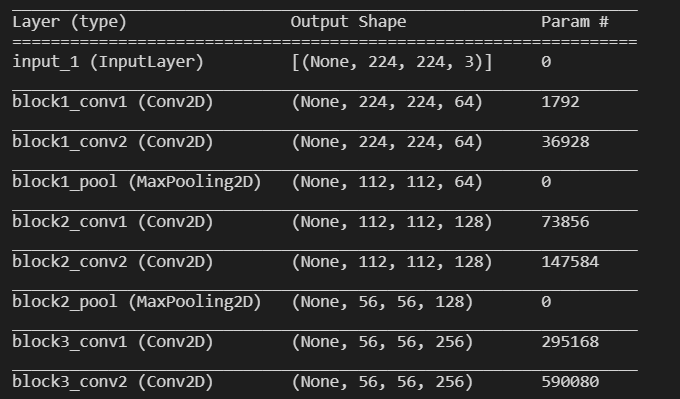举例展示第1卷积层的第1和第2特征层
plt.matshow(activations[0,:,:,0], cmap='viridis') #第1卷积层的第1特征层输出
plt.matshow(activations[0,:,:,1], cmap='viridis') #第1卷积层的第0特征层输出

可以看出第1特征层主要捕获了猫星人的轮廓特征，第2特征层主要捕获毛脸部的特征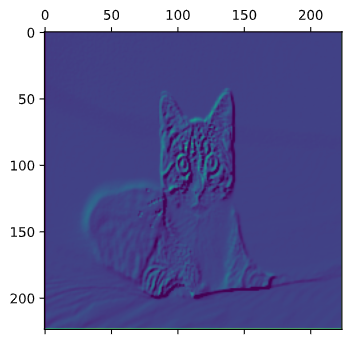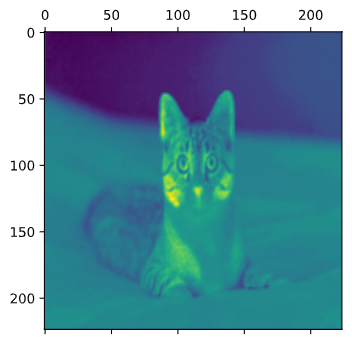所有中间层的显示
layer_names = []
for layer in VGG16_model.layers[:16]:
layer_names.append(layer.name) #特征层的名字

images_per_row=16

for layer_name, layer_activation in zip (layer_names[1:16], activations[1:16]):
n_feature = layer_activation.shape[-1] # 每层输出的特征层数
size = layer_activation.shape  #每层的特征大小
n_cols = n_feature//images_per_row #特征图平铺的行数
display_grid = np.zeros((size*n_cols, images_per_row*size)) # 每层图片大小
for col in range(n_cols): #行扫描
for row in  range (images_per_row): #平铺每行
# print(layer_activation.shape)
# print(col*images_per_row+row)
channel_image = layer_activation[0,:,:,col*images_per_row+row] # 写入col*images_per_row+row特征层
channel_image -= channel_image.mean() #标准化处理，增加可视化效果
channel_image /= channel_image.std()
channel_image *=64
channel_image +=128
channel_image = np.clip(channel_image, 0, 255).astype('uint8')
# print(channel_image.shape)
# print(display_grid[col*size:(col+1)*size, row*size:(row+1)*size].shape)
display_grid[col*size:(col+1)*size, row*size:(row+1)*size] = channel_image #写入大图中
scale = 1./size #每组图缩放系数
plt.figure(figsize=(scale*display_grid.shape, scale*display_grid.shape))
plt.title(layer_name)
plt.grid(False)
plt.imshow(display_grid, aspect='auto', cmap='viridis')


康康结果，确实符合随着层数的加深，激活变得越来越抽象，以及激活的稀疏度（sparsity）随着层数的加深而增大的预期结果。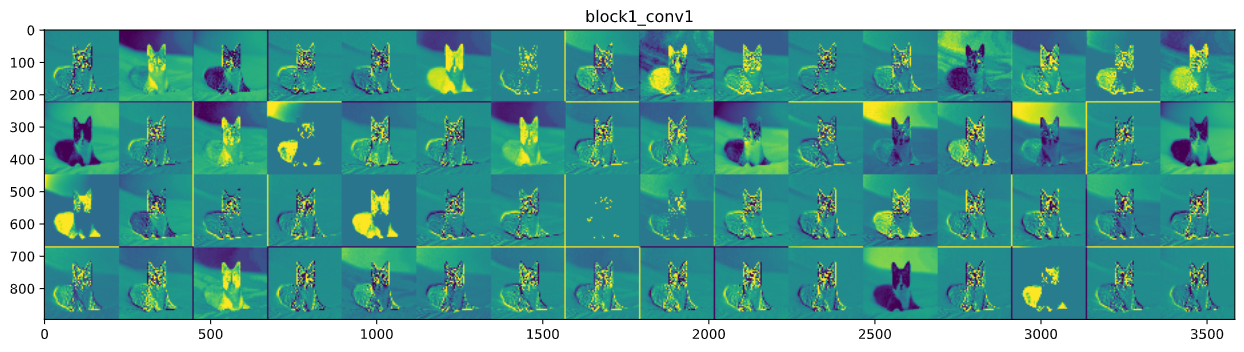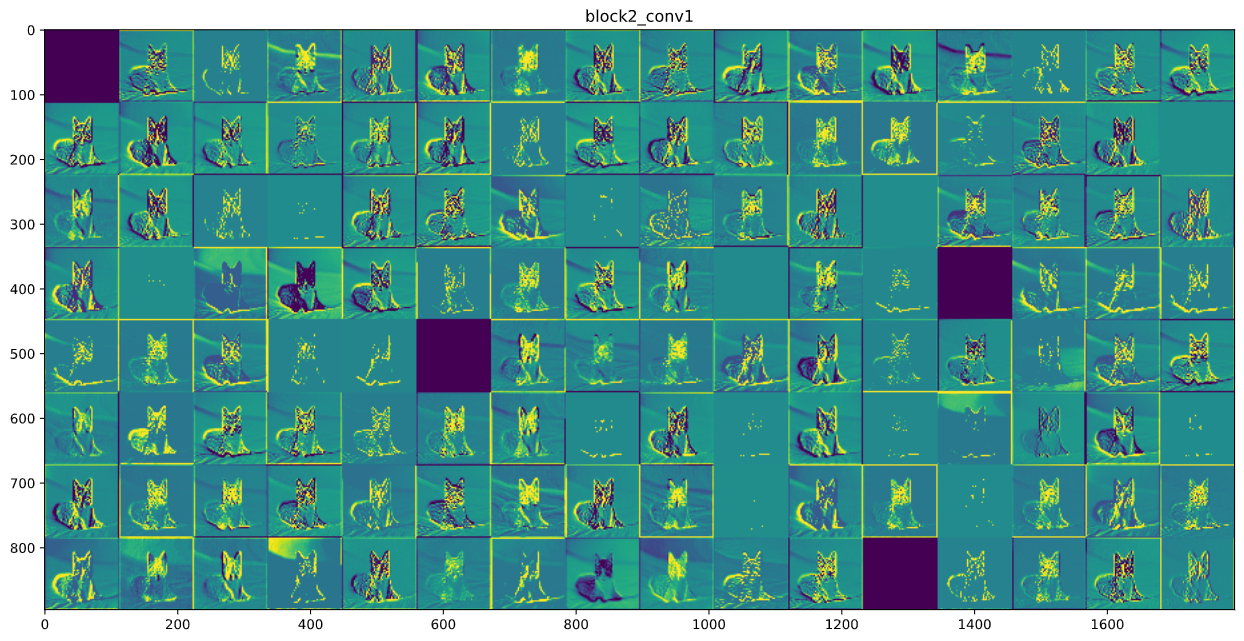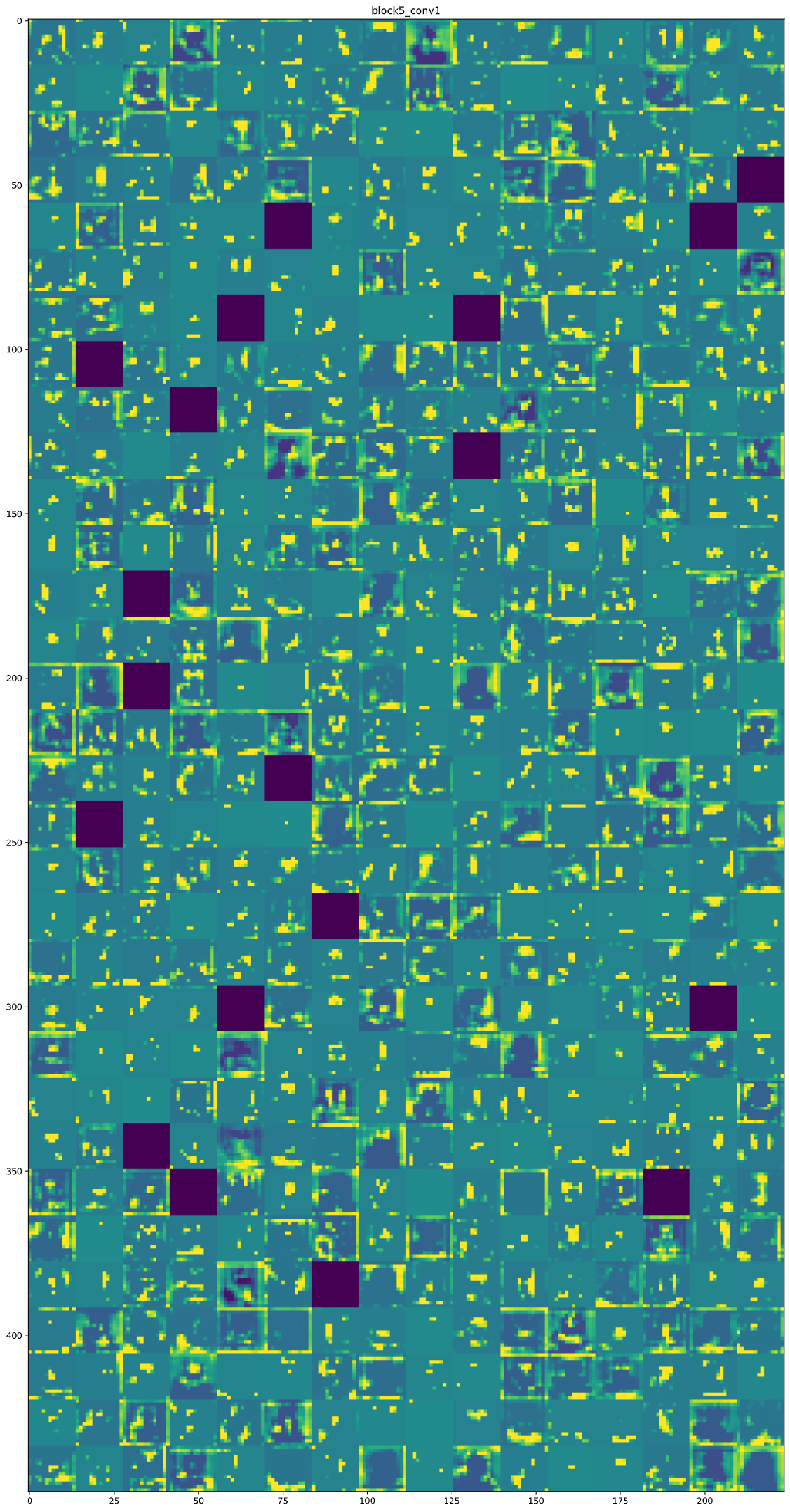参考
《Deep Learning with Python》


展开全文深度学习 tensorflow
• 本文主要是实现了个简单的卷积神经网络,并对卷积过程中的提取特征进行了可视化. 卷积神经网络最早是为了解决图像识别的问题,现在也用在时间序列数据和文本数据处理当中,卷积神经网络对于数据特征的提取不用额外...
版权声明：本文为博主原创文章，未经博主允许不得转载。
本文主要是实现了一个简单的卷积神经网络,并对卷积过程中的提取特征进行了可视化.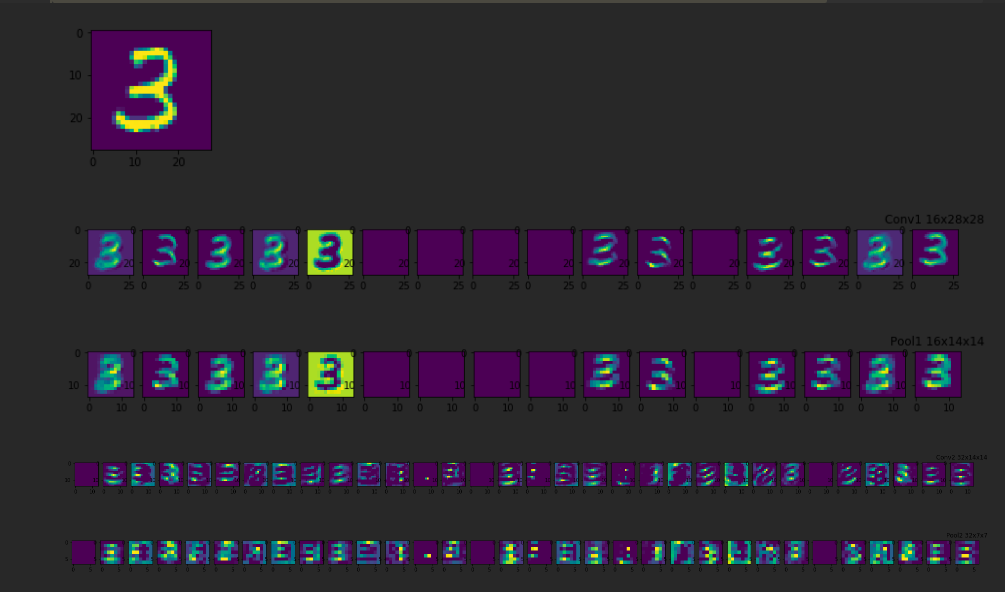卷积神经网络最早是为了解决图像识别的问题,现在也用在时间序列数据和文本数据处理当中,卷积神经网络对于数据特征的提取不用额外进行,在对网络的训练的过程当中,网络会自动提取主要的特征.
卷积神经网络直接用原始图像的全部像素作为输入,但是内部为非全连接结构.因为图像数据在空间上是有组织结构的,每一个像素在空间上和周围的像素是有关系的,和相距很远的像素基本上是没什么联系的,每个神经元只需要接受局部的像素作为输入,再将局部信息汇总就能得到全局信息.
权值共享和池化两个操作使网络模型的参数大幅的减少,提高了模型的训练效率.
权值共享:
在卷积层中可以有多个卷积核,每个卷积核与原始图像进行卷积运算后会映射出一个新的2D图像,新图像的每个像素都来自同一个卷积核.这就是权值共享.
池化:
降采样,对卷积(滤波)后,经过激活函数处理后的图像,保留像素块中灰度值最高的像素点(保留最主要的特征),比如进行 2X2的最大池化,把一个2x2的像素块降为1x1的像素块.
# 卷积网络的训练数据为MNIST(28*28灰度单色图像)
import tensorflow as tf
import numpy as np
import matplotlib.pyplot as plt
from tensorflow.examples.tutorials.mnist import input_data


训练参数
train_epochs = 100    # 训练轮数
batch_size   = 100     # 随机出去数据大小
display_step = 1       # 显示训练结果的间隔
learning_rate= 0.0001  # 学习效率
drop_prob    = 0.5     # 正则化,丢弃比例
fch_nodes    = 512     # 全连接隐藏层神经元的个数

网络结构
[外链图片转存失败,源站可能有防盗链机制,建议将图片保存下来直接上传(img-GPwo1L58-1579072744365)(output_16_11.png)]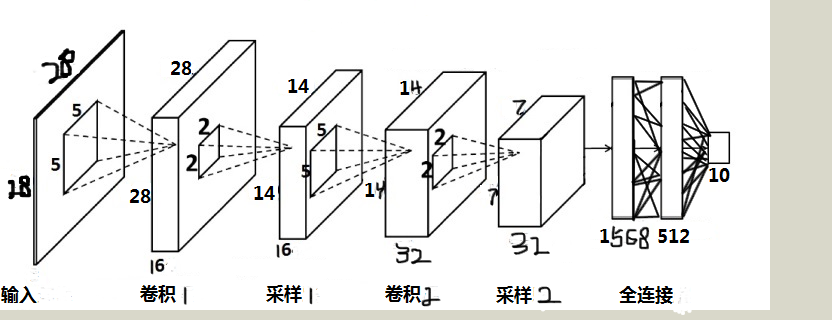输入层为输入的灰度图像尺寸:  -1 x 28 x 28 x 1
第一个卷积层,卷积核的大小,深度和数量 (5, 5, 1, 16)
池化后的特征张量尺寸:       -1 x 14 x 14 x 16
第二个卷积层,卷积核的大小,深度和数量 (5, 5, 16, 32)
池化后的特征张量尺寸:       -1 x 7 x 7 x 32
全连接层权重矩阵         1568 x 512
输出层与全连接隐藏层之间,  512 x 10

一些辅助函数
# 网络模型需要的一些辅助函数
# 权重初始化(卷积核初始化)
# tf.truncated_normal()不同于tf.random_normal(),返回的值中不会偏离均值两倍的标准差
# 参数shpae为一个列表对象,例如[5, 5, 1, 32]对应
# 5,5 表示卷积核的大小, 1代表通道channel,对彩色图片做卷积是3,单色灰度为1
# 最后一个数字32,卷积核的个数,(也就是卷基层提取的特征数量)
#   显式声明数据类型,切记
def weight_init(shape):
weights = tf.truncated_normal(shape, stddev=0.1,dtype=tf.float32)
return tf.Variable(weights)

# 偏置的初始化
def biases_init(shape):
biases = tf.random_normal(shape,dtype=tf.float32)
return tf.Variable(biases)

# 随机选取mini_batch
def get_random_batchdata(n_samples, batchsize):
start_index = np.random.randint(0, n_samples - batchsize)
return (start_index, start_index + batchsize)

# 全连接层权重初始化函数xavier
def xavier_init(layer1, layer2, constant = 1):
Min = -constant * np.sqrt(6.0 / (layer1 + layer2))
Max = constant * np.sqrt(6.0 / (layer1 + layer2))
return tf.Variable(tf.random_uniform((layer1, layer2), minval = Min, maxval = Max, dtype = tf.float32))

# 卷积
def conv2d(x, w):
return tf.nn.conv2d(x, w, strides=[1, 1, 1, 1], padding='SAME')

# 源码的位置在tensorflow/python/ops下nn_impl.py和nn_ops.py
# 这个函数接收两个参数,x 是图像的像素, w 是卷积核
# x 张量的维度[batch, height, width, channels]
# w 卷积核的维度[height, width, channels, channels_multiplier]
# tf.nn.conv2d()是一个二维卷积函数,
# stirdes 是卷积核移动的步长,4个1表示,在x张量维度的四个参数上移动步长
# 填充,是指在原图像素值矩阵周围填充0像素点
# 如果不进行填充,假设 原图为 32x32 的图像,卷积和大小为 5x5 ,卷积后映射图像大小 为 28x28

卷积核在提取特征时的动作成为padding，它有两种方式：SAME和VALID。卷积核的移动步长不一定能够整除图片像素的宽度，所以在有些图片的边框位置有些像素不能被卷积。这种不越过边缘的取样就叫做 valid padding，卷积后的图像面积小于原图像。为了让卷积核覆盖到所有的像素，可以对边缘位置进行0像素填充，然后在进行卷积。这种越过边缘的取样是 same padding。如过移动步长为1，那么得到和原图一样大小的图像。

# 池化
def max_pool_2x2(x):
return tf.nn.max_pool(x, ksize=[1, 2, 2, 1], strides=[1, 2, 2, 1], padding='SAME')

# 池化跟卷积的情况有点类似
# x 是卷积后,有经过非线性激活后的图像,
# ksize 是池化滑动张量
# ksize 的维度[batch, height, width, channels],跟 x 张量相同
# strides [1, 2, 2, 1],与上面对应维度的移动步长

# x 是手写图像的像素值,y 是图像对应的标签
x = tf.placeholder(tf.float32, [None, 784])
y = tf.placeholder(tf.float32, [None, 10])
# 把灰度图像一维向量,转换为28x28二维结构
x_image = tf.reshape(x, [-1, 28, 28, 1])
# -1表示任意数量的样本数,大小为28x28深度为一的张量
# 可以忽略(其实是用深度为28的,28x1的张量,来表示28x28深度为1的张量)

第一层卷积+池化

w_conv1 = weight_init([5, 5, 1, 16])                             # 5x5,深度为1,16个
b_conv1 = biases_init()
h_conv1 = tf.nn.relu(conv2d(x_image, w_conv1) + b_conv1)    # 输出张量的尺寸:28x28x16
h_pool1 = max_pool_2x2(h_conv1)                                   # 池化后张量尺寸:14x14x16
# h_pool1 , 14x14的16个特征图

第二层卷积+池化

w_conv2 = weight_init([5, 5, 16, 32])                             # 5x5,深度为16,32个
b_conv2 = biases_init()
h_conv2 = tf.nn.relu(conv2d(h_pool1, w_conv2) + b_conv2)    # 输出张量的尺寸:14x14x32
h_pool2 = max_pool_2x2(h_conv2)                                   # 池化后张量尺寸:7x7x32
# h_pool2 , 7x7的32个特征图

全连接层
# h_pool2是一个7x7x32的tensor,将其转换为一个一维的向量
h_fpool2 = tf.reshape(h_pool2, [-1, 7*7*32])
# 全连接层,隐藏层节点为512个
# 权重初始化
w_fc1 = xavier_init(7*7*32, fch_nodes)
b_fc1 = biases_init([fch_nodes])
h_fc1 = tf.nn.relu(tf.matmul(h_fpool2, w_fc1) + b_fc1)

# 全连接隐藏层/输出层
# 为了防止网络出现过拟合的情况,对全连接隐藏层进行 Dropout(正则化)处理,在训练过程中随机的丢弃部分
# 节点的数据来防止过拟合.Dropout同把节点数据设置为0来丢弃一些特征值,仅在训练过程中,
# 预测的时候,仍使用全数据特征
# 传入丢弃节点数据的比例
#keep_prob = tf.placeholder(tf.float32)
h_fc1_drop = tf.nn.dropout(h_fc1, keep_prob=drop_prob)

# 隐藏层与输出层权重初始化
w_fc2 = xavier_init(fch_nodes, 10)
b_fc2 = biases_init()

# 未激活的输出
# 激活后的输出
y_out = tf.nn.softmax(y_)

# 交叉熵代价函数
cross_entropy = tf.reduce_mean(-tf.reduce_sum(y * tf.log(y_out), reduction_indices = ))

# tensorflow自带一个计算交叉熵的方法
# 输入没有进行非线性激活的输出值 和 对应真实标签
#cross_loss = tf.reduce_mean(tf.nn.softmax_cross_entropy_with_logits(y_, y))

# 准确率
# 每个样本的预测结果是一个(1,10)的vector
correct_prediction = tf.equal(tf.argmax(y, 1), tf.argmax(y_out, 1))
# tf.cast把bool值转换为浮点数
accuracy = tf.reduce_mean(tf.cast(correct_prediction, tf.float32))

# 全局变量进行初始化的Operation
init = tf.global_variables_initializer()

# 加载数据集MNIST
n_samples = int(mnist.train.num_examples)
total_batches = int(n_samples / batch_size)

# 会话
with tf.Session() as sess:
sess.run(init)
Cost = []
Accuracy = []
for i in range(train_epochs):

for j in range(100):
start_index, end_index = get_random_batchdata(n_samples, batch_size)

batch_x = mnist.train.images[start_index: end_index]
batch_y = mnist.train.labels[start_index: end_index]
_, cost, accu = sess.run([ optimizer, cross_entropy,accuracy], feed_dict={x:batch_x, y:batch_y})
Cost.append(cost)
Accuracy.append(accu)
if i % display_step ==0:
print ('Epoch : %d ,  Cost : %.7f'%(i+1, cost))
print 'training finished'
# 代价函数曲线
fig1,ax1 = plt.subplots(figsize=(10,7))
plt.plot(Cost)
ax1.set_xlabel('Epochs')
ax1.set_ylabel('Cost')
plt.title('Cross Loss')
plt.grid()
plt.show()
# 准确率曲线
fig7,ax7 = plt.subplots(figsize=(10,7))
plt.plot(Accuracy)
ax7.set_xlabel('Epochs')
ax7.set_ylabel('Accuracy Rate')
plt.title('Train Accuracy Rate')
plt.grid()
plt.show()
#----------------------------------各个层特征可视化-------------------------------
# imput image
fig2,ax2 = plt.subplots(figsize=(2,2))
ax2.imshow(np.reshape(mnist.train.images, (28, 28)))
plt.show()

# 第一层的卷积输出的特征图
input_image = mnist.train.images[11:12]
conv1_16 = sess.run(h_conv1, feed_dict={x:input_image})     # [1, 28, 28 ,16]
conv1_transpose = sess.run(tf.transpose(conv1_16, [3, 0, 1, 2]))
fig3,ax3 = plt.subplots(nrows=1, ncols=16, figsize = (16,1))
for i in range(16):
ax3[i].imshow(conv1_transpose[i])                      # tensor的切片[row, column]

plt.title('Conv1 16x28x28')
plt.show()

# 第一层池化后的特征图
pool1_16 = sess.run(h_pool1, feed_dict={x:input_image})     # [1, 14, 14, 16]
pool1_transpose = sess.run(tf.transpose(pool1_16, [3, 0, 1, 2]))
fig4,ax4 = plt.subplots(nrows=1, ncols=16, figsize=(16,1))
for i in range(16):
ax4[i].imshow(pool1_transpose[i])

plt.title('Pool1 16x14x14')
plt.show()

# 第二层卷积输出特征图
conv2_32 = sess.run(h_conv2, feed_dict={x:input_image})          # [1, 14, 14, 32]
conv2_transpose = sess.run(tf.transpose(conv2_32, [3, 0, 1, 2]))
fig5,ax5 = plt.subplots(nrows=1, ncols=32, figsize = (32, 1))
for i in range(32):
ax5[i].imshow(conv2_transpose[i])
plt.title('Conv2 32x14x14')
plt.show()

# 第二层池化后的特征图
pool2_32 = sess.run(h_pool2, feed_dict={x:input_image})         #[1, 7, 7, 32]
pool2_transpose = sess.run(tf.transpose(pool2_32, [3, 0, 1, 2]))
fig6,ax6 = plt.subplots(nrows=1, ncols=32, figsize = (32, 1))
plt.title('Pool2 32x7x7')
for i in range(32):
ax6[i].imshow(pool2_transpose[i])

plt.show()


Epoch : 1 ,  Cost : 1.7629557
Epoch : 2 ,  Cost : 0.8955871
Epoch : 3 ,  Cost : 0.6002768
Epoch : 4 ,  Cost : 0.4222347
Epoch : 5 ,  Cost : 0.4106165
Epoch : 6 ,  Cost : 0.5070749
Epoch : 7 ,  Cost : 0.5032627
Epoch : 8 ,  Cost : 0.3399751
Epoch : 9 ,  Cost : 0.1524799
Epoch : 10 ,  Cost : 0.2328545
Epoch : 11 ,  Cost : 0.1815660
Epoch : 12 ,  Cost : 0.2749544
Epoch : 13 ,  Cost : 0.2539429
Epoch : 14 ,  Cost : 0.1850740
Epoch : 15 ,  Cost : 0.3227096
Epoch : 16 ,  Cost : 0.0711472
Epoch : 17 ,  Cost : 0.1688010
Epoch : 18 ,  Cost : 0.1442217
Epoch : 19 ,  Cost : 0.2415594
Epoch : 20 ,  Cost : 0.0848383
Epoch : 21 ,  Cost : 0.1879225
Epoch : 22 ,  Cost : 0.1355369
Epoch : 23 ,  Cost : 0.1578972
Epoch : 24 ,  Cost : 0.1017473
Epoch : 25 ,  Cost : 0.2265745
Epoch : 26 ,  Cost : 0.2625684
Epoch : 27 ,  Cost : 0.1950202
Epoch : 28 ,  Cost : 0.0607868
Epoch : 29 ,  Cost : 0.0782418
Epoch : 30 ,  Cost : 0.0744723
Epoch : 31 ,  Cost : 0.0848689
Epoch : 32 ,  Cost : 0.1038134
Epoch : 33 ,  Cost : 0.0848786
Epoch : 34 ,  Cost : 0.1219746
Epoch : 35 ,  Cost : 0.0889094
Epoch : 36 ,  Cost : 0.0605406
Epoch : 37 ,  Cost : 0.0478896
Epoch : 38 ,  Cost : 0.1100840
Epoch : 39 ,  Cost : 0.0168766
Epoch : 40 ,  Cost : 0.0479708
Epoch : 41 ,  Cost : 0.1187883
Epoch : 42 ,  Cost : 0.0707371
Epoch : 43 ,  Cost : 0.0471128
Epoch : 44 ,  Cost : 0.1206998
Epoch : 45 ,  Cost : 0.0674985
Epoch : 46 ,  Cost : 0.1218394
Epoch : 47 ,  Cost : 0.0840694
Epoch : 48 ,  Cost : 0.0468497
Epoch : 49 ,  Cost : 0.0899443
Epoch : 50 ,  Cost : 0.0111846
Epoch : 51 ,  Cost : 0.0653627
Epoch : 52 ,  Cost : 0.1446207
Epoch : 53 ,  Cost : 0.0320902
Epoch : 54 ,  Cost : 0.0792156
Epoch : 55 ,  Cost : 0.1250363
Epoch : 56 ,  Cost : 0.0477339
Epoch : 57 ,  Cost : 0.0249218
Epoch : 58 ,  Cost : 0.0571465
Epoch : 59 ,  Cost : 0.0152223
Epoch : 60 ,  Cost : 0.0373616
Epoch : 61 ,  Cost : 0.0417238
Epoch : 62 ,  Cost : 0.0710011
Epoch : 63 ,  Cost : 0.0654174
Epoch : 64 ,  Cost : 0.0234730
Epoch : 65 ,  Cost : 0.0267291
Epoch : 66 ,  Cost : 0.0329132
Epoch : 67 ,  Cost : 0.0344089
Epoch : 68 ,  Cost : 0.1151591
Epoch : 69 ,  Cost : 0.0555586
Epoch : 70 ,  Cost : 0.0213475
Epoch : 71 ,  Cost : 0.0567649
Epoch : 72 ,  Cost : 0.1207196
Epoch : 73 ,  Cost : 0.0407380
Epoch : 74 ,  Cost : 0.0580697
Epoch : 75 ,  Cost : 0.0352901
Epoch : 76 ,  Cost : 0.0420529
Epoch : 77 ,  Cost : 0.0016548
Epoch : 78 ,  Cost : 0.0184542
Epoch : 79 ,  Cost : 0.0657262
Epoch : 80 ,  Cost : 0.0185127
Epoch : 81 ,  Cost : 0.0211956
Epoch : 82 ,  Cost : 0.0709701
Epoch : 83 ,  Cost : 0.1013358
Epoch : 84 ,  Cost : 0.0876017
Epoch : 85 ,  Cost : 0.1351897
Epoch : 86 ,  Cost : 0.1239478
Epoch : 87 ,  Cost : 0.0147001
Epoch : 88 ,  Cost : 0.0155131
Epoch : 89 ,  Cost : 0.0425102
Epoch : 90 ,  Cost : 0.0912542
Epoch : 91 ,  Cost : 0.0445287
Epoch : 92 ,  Cost : 0.0823120
Epoch : 93 ,  Cost : 0.0155016
Epoch : 94 ,  Cost : 0.0869377
Epoch : 95 ,  Cost : 0.0641734
Epoch : 96 ,  Cost : 0.0498264
Epoch : 97 ,  Cost : 0.0289681
Epoch : 98 ,  Cost : 0.0271511
Epoch : 99 ,  Cost : 0.0131940
Epoch : 100 ,  Cost : 0.0418167
training finished

训练交叉熵代价
[外链图片转存失败,源站可能有防盗链机制,建议将图片保存下来直接上传(img-PYsKPfAn-1579072744371)(output_16_1.png)]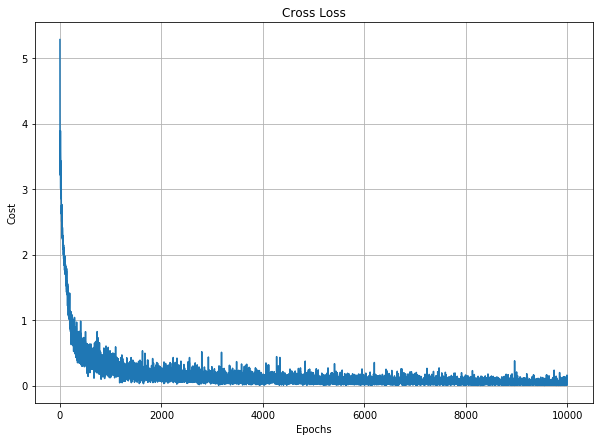训练的准确率
[外链图片转存失败,源站可能有防盗链机制,建议将图片保存下来直接上传(img-Tid5efaN-1579072744374)(output_16_2.png)]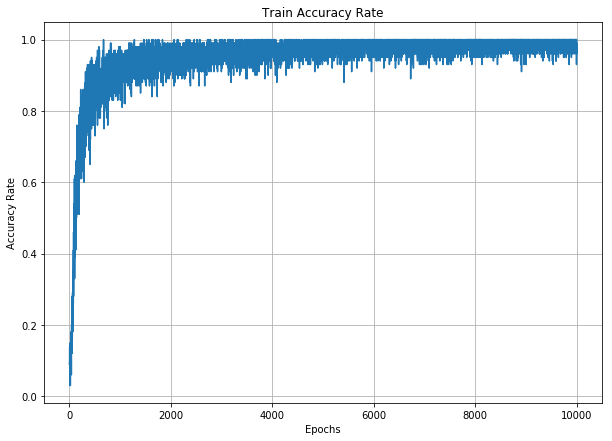训练数据中的一个样本
[外链图片转存失败,源站可能有防盗链机制,建议将图片保存下来直接上传(img-akgag6qr-1579072744380)(output_16_3.png)]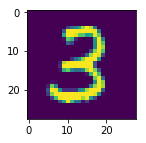第一个卷积层提取的特征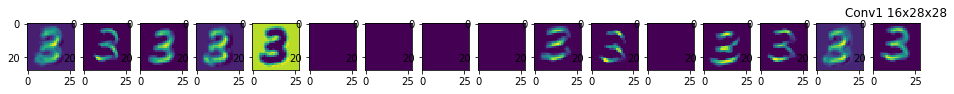2x2池化后的特征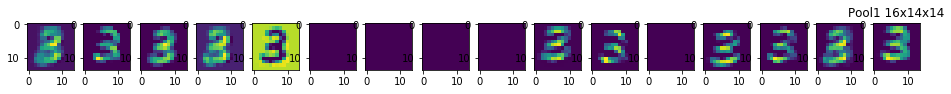第二层卷积提取特征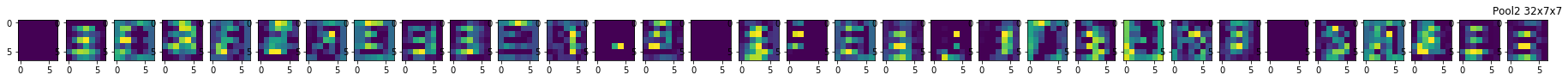2x2池化后的特征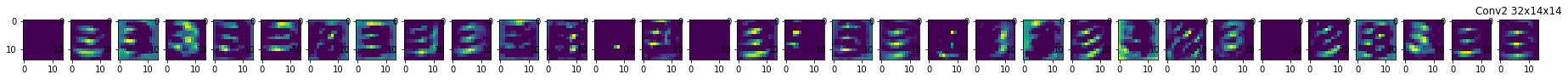安利一下，公众号展开全文• 卷积神经网络可视化可视化卷积神经网络中间输出（中间激活），可视化卷积神经网络的过滤器，可视化图像中类激活的热力图。 可视化中间激活：对于指定输入，展示网络中各个卷积层和池化层输出的特征图（层的输出...
卷积神经网络的可视化：可视化卷积神经网络中间输出（中间激活），可视化卷积神经网络的过滤器，可视化图像中类激活的热力图。

可视化中间激活：对于指定输入，展示网络中各个卷积层和池化层输出的特征图（层的输出通常叫做层的激活）
特征图中，第一层是更重边缘探测器的集合，随着层数加深，激活变得越来越抽象，激活的稀疏度随着层数的加深而增大。

深度神经网络可以有效的作为信息蒸馏管道，输入原始数据，反复对其进行变换，过滤无关信息，放大和细化有用的信息。

from keras.models import load_model
model.summary()

#预处理单张图像
img_path='D:\\jupyter_code\\kaggle\\train1\\test\\cats\\cat.1700.jpg'
from keras.preprocessing import image
import numpy as np
#图像预处理为一个4D张量
#将python图像库转换为一个numpy数组
img_tensor=image.img_to_array(img)
#拓展转换后的数组形状，完成4D张量的转换
img_tensor=np.expand_dims(img_tensor,axis=0)
img_tensor/=255.

print(img_tensor.shape)

import matplotlib.pyplot as plt
plt.imshow(img_tensor)
plt.show()

#用输入输出张量列表将模型实例化，（图像批量作为输入，输出所有卷积层的激活）
from keras import models
#提取前8层的输出
layer_outputs=[layer.output for layer in model.layers[:8]]
activation_model=models.Model(inputs=model.input,outputs=layer_outputs)
#返回8个Numpy数组组成的列表，每个层对应激活一个numpy数组。
activations=activation_model.predict(img_tensor)

first_layer_activation=activations
print(first_layer_activation.shape)
#打印特征图形状可以看到有32个通道，每层对应4个通道

#将第7个通道可视化
import matplotlib.pyplot as plt
plt.matshow(first_layer_activation[0, :,:,7],cmap='viridis')

#将每个中间激活的通道可视化
#为层添加
layer_names=[]
for layer in model.layers[:8]:
layer_names.append(layer.name)

images_per_row=16
#显示特征图
for layer_names,layer_activation in zip(layer_names,activations):
#特征图中特征格式
n_feature=layer_activation.shape[-1]
#特征形状
size=layer_activation.shape
n_cols=n_feature//images_per_row
display_grid=np.zeros((size*n_cols,images_per_row*size))
#将过滤器平铺到打的水平网络
for col in range(n_cols):
for row in range(images_per_row):
channel_image=layer_activation[0,:,:,col*images_per_row+row]
channel_image-=channel_image.mean()
channel_image/=channel_image.std()
channel_image*=64
channel_image+=128
channel_image=np.clip(channel_image,0,255).astype('uint8')
display_grid[col*size:(col+1)*size,
row*size:(row+1)*size]=channel_image
scale=1./size
plt.figure(figsize=(scale*display_grid.shape,
scale*display_grid.shape))
plt.title(layer_names)
plt.grid(False)
plt.imshow(display_grid,aspect='auto',cmap='viridis')

2.可视化卷积神经网络的过滤器（显示过滤器响应的视觉模式）

显示每个过滤器所响应的视觉模式，通过在输入空间中进行梯度上升来实现：从空白输入图像开始，将梯度下降应用于卷积神经网络输入图像的值，目的是让某个过滤器的响应最大化。得到的输入图像是选定过滤器具有最大响应的图像。

卷积神经网络中每一层都设置一组过滤器，以便其输入表示为过滤器的组合。随着层数加深，卷积神经网络中过滤变得越来越复杂，越来越精细。

#可视化卷积神经网络的过滤器

#为过滤器的可视化定义损失张量
from keras.applications import VGG16
#此处的k为TensorFlow
from keras import backend as K

model=VGG16(weights='imagenet',include_top=False)

layer_name='block3_conv1'
filter_index=0

layer_output=model.get_layer(layer_name).output
loss=K.mean(layer_output[:,:,:,filter_index])
#梯度标准化技巧

#给定Numpy输入值，得到Numpy输出值
#iterare将一个Numpy张量转换为2个Numpy张量组成的列表
import numpy as np

#通过随机梯度下降让损失最大化
input_img_data=np.random.random((1,150,150,3))*20+128
#每次梯度更新的步长
step=1.
for i in range(40):
#计算损失值和梯度值
#沿着让损失最大化的方向调节输入图像（梯度上升）

#将张量转换为有效图像的使用函数
def deprocess_image(x):
#张量标准化，均值为0 ，标准差为0.1
x-=x.mean()
x/=(x.std()+1e-5)
x*=0.1
#将x裁剪到（0，1）区间
x+=0.5
x=np.clip(x,0,1)
#x转RGB数组
x*=255
x=np.clip(x,0,255).astype('uint8')
return x

#生成过滤器的可视化函数
def generate_pattern(layer_name,filter_index,size=150):
#构建损失函数，该层第n个过滤器的激活最大化
layer_output=model.get_layer(layer_name).output
loss=K.mean(layer_output[:,:,:,filter_index])
#计算损失相对于输入图像的梯度
#梯度标准化
#返回给定输入图像的损失和梯度

input_img_data=np.random.random((1,size,size,3))*20+128
#运行40次梯度上升
step=1.
for i in range(40):

img=input_img_data
return deprocess_image(img)

plt.imshow(generate_pattern('block3_conv1',0))

layer_name='block2_conv1'
size=64
margin=5

results=np.zeros((8*size+7*margin,8*size+7*margin,3))

for i in range(8):
for j in range(8):
filter_img=generate_pattern(layer_name,i+(j*8),size=size)

horizontal_start=i*size+i*margin
horizontal_end=horizontal_start+size
vertical_start=j*size+j*margin
vertical_end=vertical_start+size

results[horizontal_start:horizontal_end,vertical_start:vertical_end,:]=filter_img

plt.figure(figsize=(20,20))
plt.imshow(results)

3.可视化类激活的热力图（了解一张图像的那一部分让神经网络做出了分类决策）

类激活图可视化:类激活热力图是与特定输出类别相关的二维分数网络，对任何输入图像的每个位置都要进行计算，表示每个位置对类别的重要程度。

实现方法：给定输入图像，对于卷积层的输出特征图，用类别相对于通道的梯度对这个特征图这个每个通道进行加权。

由“每个通道对类别的重要程度”对 “不同通道的激活程度”的空间图加权，得到“类别的激活程度”（A->B）&(A->C）à(B->C)

展开全文python 读书笔记
• 之所以称之卷积神经网络，是因为，隐藏层中使用了卷积层，来处理二（灰度）或三（RGB）的图像数据。每个卷积层由多个过滤器(Filter)组成，每个过滤器对应个小矩阵（行列数通常为2或者3），矩阵沿着图像的行列...图像卷积
• ## 【keras】一维卷积神经网络多分类

万次阅读 多人点赞 2020-06-20 13:05:05
刚刚接触到深度学习，前2个月的时间里，我用一维卷积神经网络实现了对于一维数据集的分类和回归。由于在做这次课题之前，我对深度学习基本上没有过接触，所以期间走了很多弯路。 在刚刚收到题目的要求时，我选择...深度学习 分类算法 python
• 卷积神经网络学到的表示非常适合可视化，很大程度上是因为它们 是视觉概念的表示 ''' #可视化中间激活： ''' #对于给定输入，展示网络中各个卷积层和池化层输出的特征图（ 层的输出通常被称为该层的激活，即激活...
• 可视化最大激活神经元的一些pathces 例如在这个AlexNet的pool5层任选神经元，然后喂给它大量的图片，看哪种图片最能激活该神经元 比如上面两行代表使神经元激活值最大的一些图片，白框是感受野，数值即...
• 神经网络分别中，我们不仅想知道最终预测结果，还需要了解网络是凭借图像什么特征进行判断的。其中类激活热力图 (CAM，class activation map）就是种很好的呈现方式。 目录标题类激活热力图 (CAM，class ...深度学习 tensorflow
• 1.keras可视化深度网络各层特征；2.ＣＮＮ原理keras CNN原理
• 基于一维卷积神经网络的滚动轴承故障识别 提示：文章写完后，目录可以自动生成，如何生成可参考右边的帮助文档 文章目录基于一维卷积神经网络的滚动轴承故障识别一、数据预处理二、模型搭建三、使用步骤1.引入库2....深度学习
• #下面这个我得电脑打不起来，程序下面附有修改过的可视化程序# coding: utf-8 # In: import tensorflow as tf from tensorflow.examples.tutorials.mnist import input_data # In: mnist = input_data....
• 可视化卷积神经网络的中间输出（中间激活）：有助于理解卷积神经网络连续的层如何对输入进行变换，也有助于初步了解卷积神经网络每个过滤器的含义。 二、可视化卷积神经网络的过滤器：有助于精确理解卷积神经...
• 卷积神经网络可视化中间激活 **摘要：**卷积神经网络学到的表示非常适合可视化，很大程度是因为它们是视觉概念的表示。本文将介绍种非常容易理解也非常有用的方法—可视化中间激活。可视化中间激活，是指对于...列表 机器学习
• 使用Tensorflow和vgg16预训练好的模型实现了卷积神经网络中特征图（feature map）的可视化，可以更明了的知道这个黑箱中到底发生了什么。 卷积神经网络特征图可视化 代码如下： # -*- coding:utf-8 -*- import ...tensorflow python
• 关注上方“深度学习技术前沿”，选择“星标公众号”，资源干货，第时间送达！来源：量子位CNN是什么？美国有线电视新闻网吗？每个对AI抱有憧憬的小白，在开始的时候都会遇到CNN（卷积神经...
• 如果我们想要观察神经网络中任意个神经元到底对何种图片响应最大（激活值最大），通常做法是： 、不用正则项 首先随机为每个像素赋值，得到个随机图片，前向传播算出对于网络中某个神经元i的激活值ai(x),...
• 但是我们现在的学习的卷积伸进网络却可以通过可视化很形象的说明深度学习在卷积神经网络方面可以很表示的，并不是黑盒。目前比较容易理解的三种可视化的方法如下： 可视化卷积神经网络的中间输出（中间激活） ...深度学习 激活函数
• 前言 在大部分人看来，卷积神经网络是种黑盒技术，通过理论推导，以及梯度传播，去不断逼近局部最优解。这也导致人们对于神经网络研究进展...今天就要为大家介绍卷积神经网络可视化的技巧，它发表于篇论文，...
• 基于1DCNN(一维卷积神经网络）的机械振动故障诊断 机械振动故障诊断最为经典的还是凯斯西储实验室的轴承故障诊断，开学一周了，上次改编鸢尾花分类的代码可用，但是并不准确。开学一周重新改编了别人的一篇代码，亲...深度学习 tensorflow
• Tensorboard是TensorFlow自带的可视化工具，因为想知道神经网络的中间环节到底是如何变化的，比如损失函数的变化过程、参数的分布、参数更新的过程、卷积核的样子等等，所以需要用到Tensorboard来帮助我。...Tensorflow
• 卷积神经网络实现图像识别及过程可视化 本博文提供经典的卷积神经网络实现代码，将CNN的工具类等代码分别封装，并提供接口自行替换使用的模型（可以换成自己的神经网络及图片样本），代码中提供模型保存和读取...深度学习 tensorflow 图像识别
• 通常我们认为深度学习模型是“黑盒”的，即模型学到的表示...1）可视化卷积神经网络的中间输出（中间激活） 有助于理解卷积网络神经连续的层如何对输入进行变换，也有助于初步了解卷积神经网络每个过滤器的含义。 ...
• 我们将介绍另可视化技术，它有助于理解给定图像的哪些部分引导convnet做出最终的分类决策。这有助于“调试”convnet的决策过程，特别是在分类错误的情况下。它还允许您在图像中定位特定对象。 这类技术被称为...python
• 卷积神经网络种特殊类型的人工神经网络，广泛应用于图像识别。这种架构的成功始于 2015 年，当时凭借这种方法赢得了 ImageNet 图像分类挑战。 这些方法非常强大并且能够很好地进行预测，但同时它们也难以解释。...深度学习 计算机视觉...